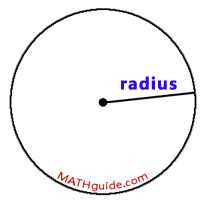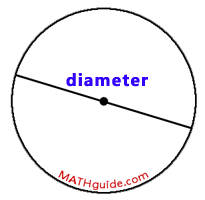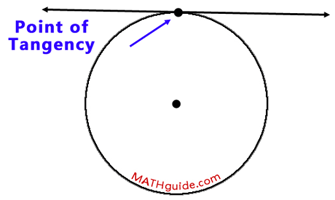Circle Terminology
 Home > Lessons > Circle Terminology Search | Updated December 2nd, 2015
Introduction
In this section of MATHguide, you will learn a variety of terms that are associated with circles. Here are the topics within this page:

 Radius We will look at geometric objects related to circles. The first object is a radius. Radius: A radius of a circle is a segment that has one endpoint on the circle and the other endpoint at the center of the circle.     A picture of a radius can be seen here.Chord The next geometric object for us to examine is a chord. Chord: A chord of a circle is a segment that has both of its endpoints on the circle.     A picture of a chord can be seen here.Diameter Our next geometric object is a diamter. Diameter: The diamter of a circle is a segment that has both of its endpoints on the circle and it passes throught the center of the circle.     A diameter is the longest chord of a circle. A picture of a diameter can be seen here.Secant Next on our list of geometric objects is a secant line. Secant: A secant is a line that passes through two points on a circle.     A picture of a secant can be seen here.Tangent The next geometric object is one that has great significance within calculus. It is a tangent. Tangent: A tangent is a line that intersects a circle only once.     A picture of a tangent can be seen here.Point of Tangency The next geometric object is called the point of tangency. Point of Tangency: The point of tangency is a point where a tangent line intersects a circle.     A picture of a point of tangency can be seen here.An interesting fact concerning point of tangencies is this: A radius drawn to a point of tangency is always perpendicular to the tangent line. This can be seen within the next diagram.Instructional Videos There are no videos at this time. Interactive Quizzes There are no quizmasters at this time. Activities There are no activities at this time. Related Lessons Here are related lessons to the circle terminology.esson: Central Anglesesson: Inscribed Anglesesson: Chord-Chord Relationshipsesson: Secant-Secant Relationships